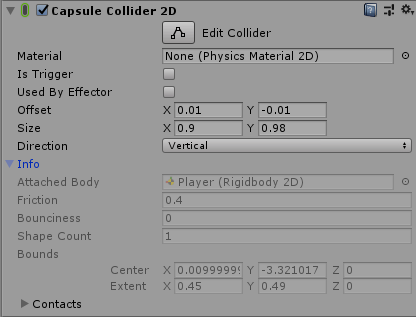# CapsuleCollider2D.bounds empty

Whenever I try debuggging the following code, my bounds appear to be empty. The center, min, and max fields are all populated with the same values, and the size and extents are all 0.

``````private Collider2D On() { return On(colmask.bounds); }
private Collider2D On(Bounds bounds)
{	const float EPSILON = 0.015f; //Mathf.Epsilon;

return Physics2D.OverlapArea
(	new Vector2(bounds.min.x + EPSILON, bounds.min.y),
new Vector2(bounds.max.x - EPSILON, bounds.min.y - EPSILON),
/*ContactFilter2D,*/
/*results,*/ //This is where the results go on some functions, can be ignored since I only need to know that there was a collision.
appropriate_layers
);
}
``````

The properties window starkly disagrees with these values, but I’m still only able to jump once in a blue moon (apparently depending on my position, and rotation, which shouldn’t matter since I am using bounds).Finally, here’s the Update function I am calling the function from.

``````void Update ()
{	dx = Input.GetAxis("Horizontal") * speed;
/**/ if (dx > 0) Cx =  1;
else if (0 > dx) Cx = -1;

var floor_collider = On( /*BoundsColliders(gameObject)*/ );
if (floor_collider!= null)
{	var floor = floor_collider.gameObject;
if (Input.GetButtonDown("Jump")) Jump();
if(floor.tag == "Enemy" && floor.transform.position.y < y)
{	var atk = floor.GetComponent<Enemy>().vigor.Attack(attack_power);
if( Health.Atk.DIES == atk ) Destroy(floor);
}

//aligns character to the ground it is standing on
ContactPoint2D[] contacts = new ContactPoint2D;